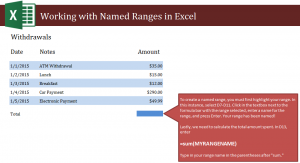# Get Sum in Excel

15
AprLearn how to get sum in Excel spreadsheets from a group of numbers using simple formulas. When working with named ranges in Excel, you’ll be able to isolate a group of numbers and then enter a formula that will tell Excel to give you the total number of all those numbers. This is a good Excel skill to know because it can save you time and effort when adding up multiple numbers in large spreadsheets.

Open your downloaded example and you will see a list of dollar amounts for withdrawals. Your goal is to get the total amount of withdrawals. To do this, you must start by creating a named range.

Select cells D7 through D11. Then, move to the upper right corner where it says “range” in a white box. Erase the word range and insert a name of your choice. For the example, we’ll use “Withdrawals”. Now cells D7 through D11 are a range named Withdrawals.

Next, to find the sum, move to cell D13 and type in this formula:

=sum(Withdrawals)

Replace Withdrawals if you named your range something else.

The cell D13 should now show the total amount in withdrawals that were made.

To practice with this process, try have two ranges and two boxes to calculate different sums. This will be helpful when working in larger Excel worksheets that require sectioning amounts.